## Equations of Ellipses

### Learning Outcomes

• Identify the foci, vertices, axes, and center of an ellipse.
• Write equations of ellipses centered at the origin.
• Write equations of ellipses not centered at the origin.

A conic section, or conic, is a shape resulting from intersecting a right circular cone with a plane. The angle at which the plane intersects the cone determines the shape.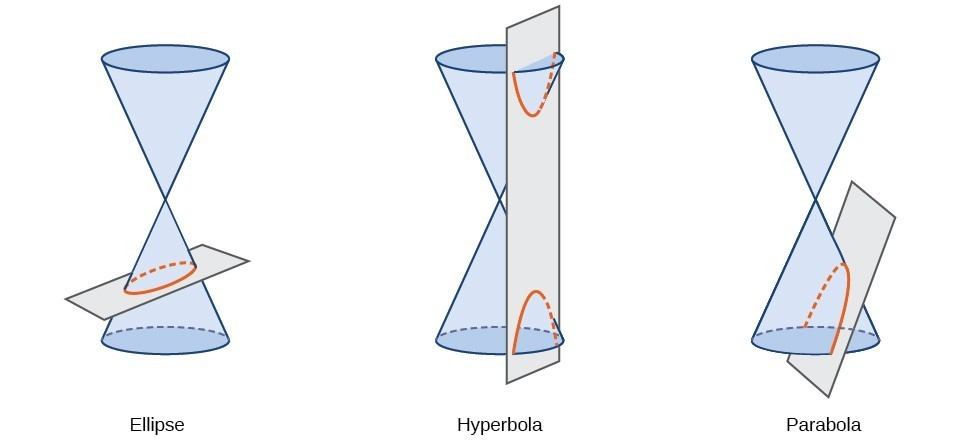Conic sections can also be described by a set of points in the coordinate plane. Later in this chapter we will see that the graph of any quadratic equation in two variables is a conic section. The signs of the equations and the coefficients of the variable terms determine the shape. This section focuses on the four variations of the standard form of the equation for the ellipse. An ellipse is the set of all points $\left(x,y\right)$ in a plane such that the sum of their distances from two fixed points is a constant. Each fixed point is called a focus (plural: foci) of the ellipse.

We can draw an ellipse using a piece of cardboard, two thumbtacks, a pencil, and string. Place the thumbtacks in the cardboard to form the foci of the ellipse. Cut a piece of string longer than the distance between the two thumbtacks (the length of the string represents the constant in the definition). Tack each end of the string to the cardboard, and trace a curve with a pencil held taut against the string. The result is an ellipse.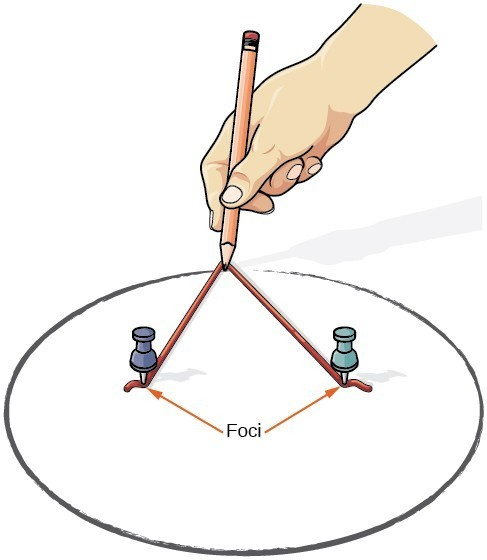Every ellipse has two axes of symmetry. The longer axis is called the major axis, and the shorter axis is called the minor axis. Each endpoint of the major axis is the vertex of the ellipse (plural: vertices), and each endpoint of the minor axis is a co-vertex of the ellipse. The center of an ellipse is the midpoint of both the major and minor axes. The axes are perpendicular at the center. The foci always lie on the major axis, and the sum of the distances from the foci to any point on the ellipse (the constant sum) is greater than the distance between the foci.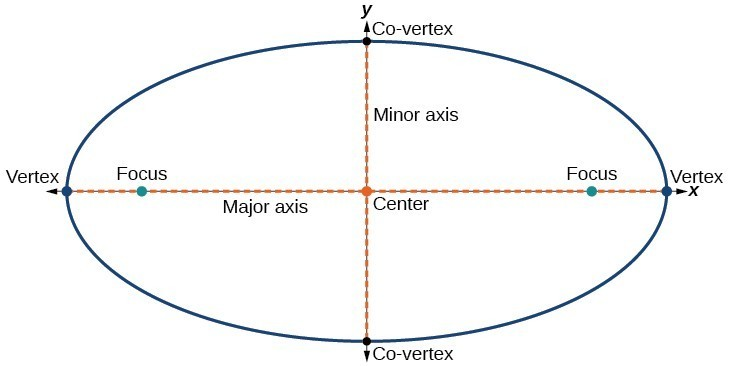In this section we restrict ellipses to those that are positioned vertically or horizontally in the coordinate plane. That is, the axes will either lie on or be parallel to the x– and y-axes. Later in the chapter, we will see ellipses that are rotated in the coordinate plane.

To work with horizontal and vertical ellipses in the coordinate plane, we consider two cases: those that are centered at the origin and those that are centered at a point other than the origin. First we will learn to derive the equations of ellipses, and then we will learn how to write the equations of ellipses in standard form. Later we will use what we learn to draw the graphs.

To derive the equation of an ellipse centered at the origin, we begin with the foci $(-c,0)$ and $(c,0)$. The ellipse is the set of all points $(x,y)$ such that the sum of the distances from $(x,y)$ to the foci is constant, as shown in the figure below.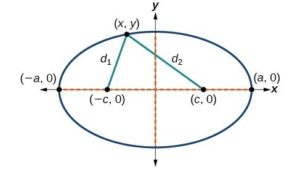If $(a,0)$ is a vertex of the ellipse, the distance from $(-c,0)$ to $(a,0)$ is $a-(-c)=a+c$. The distance from $(c,0)$ to $(a,0)$ is $a-c$. The sum of the distances from the foci to the vertex is

$(a+c)+(a-c)=2a$

If $(x,y)$ is a point on the ellipse, then we can define the following variables:

\begin{align}d_1&=\text{the distance from } (-c,0) \text{ to } (x,y) \\ d_2&= \text{the distance from } (c,0) \text{ to } (x,y) \end{align}

By the definition of an ellipse, $d_1+d_2$ is constant for any point $(x,y)$ on the ellipse. We know that the sum of these distances is $2a$ for the vertex $(a,0)$. It follows that $d_1+d_2=2a$ for any point on the ellipse. The derivation of the standard form of the equation of an ellipse relies on this relationship and the distance formula. The derivation is beyond the scope of this course, but the equation is:

$\dfrac{x^2}{a^2}+\dfrac{y^2}{b^2}=1$

for an ellipse centered at the origin with its major axis on the X-axis and

$\dfrac{x^2}{b^2}+\dfrac{y^2}{a^2}=1$

for an ellipse centered at the origin with its major axis on the Y-axis.

## Writing Equations of Ellipses Centered at the Origin in Standard Form

Standard forms of equations tell us about key features of graphs. Take a moment to recall some of the standard forms of equations we’ve worked with in the past: linear, quadratic, cubic, exponential, logarithmic, and so on. By learning to interpret standard forms of equations, we are bridging the relationship between algebraic and geometric representations of mathematical phenomena.

The key features of the ellipse are its center, verticesco-verticesfoci, and lengths and positions of the major and minor axes. Just as with other equations, we can identify all of these features just by looking at the standard form of the equation. There are four variations of the standard form of the ellipse. These variations are categorized first by the location of the center (the origin or not the origin), and then by the position (horizontal or vertical). Each is presented along with a description of how the parts of the equation relate to the graph. Interpreting these parts allows us to form a mental picture of the ellipse.

### A General Note: Standard Forms of the Equation of an Ellipse with Center $(0,0)$

The standard form of the equation of an ellipse with center $\left(0,0\right)$ and major axis parallel to the x-axis is

$\dfrac{{x}^{2}}{{a}^{2}}+\dfrac{{y}^{2}}{{b}^{2}}=1$

where

• $a>b$
• the length of the major axis is $2a$
• the coordinates of the vertices are $\left(\pm a,0\right)$
• the length of the minor axis is $2b$
• the coordinates of the co-vertices are $\left(0,\pm b\right)$
• the coordinates of the foci are $\left(\pm c,0\right)$, where ${c}^{2}={a}^{2}-{b}^{2}$.

The standard form of the equation of an ellipse with center $\left(0,0\right)$ and major axis parallel to the y-axis is

$\dfrac{{x}^{2}}{{b}^{2}}+\dfrac{{y}^{2}}{{a}^{2}}=1$

where

• $a>b$
• the length of the major axis is $2a$
• the coordinates of the vertices are $\left(0,\pm a\right)$
• the length of the minor axis is $2b$
• the coordinates of the co-vertices are $\left(\pm b,0\right)$
• the coordinates of the foci are $\left(0,\pm c\right)$, where ${c}^{2}={a}^{2}-{b}^{2}$.

Note that the vertices, co-vertices, and foci are related by the equation $c^2=a^2-b^2$. When we are given the coordinates of the foci and vertices of an ellipse, we can use the relationship to find the equation of the ellipse in standard form.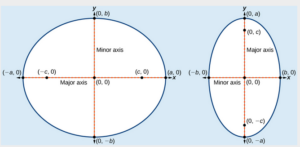Figure:                  (a) Horizontal ellipse with center (0,0),                                           (b) Vertical ellipse with center (0,0)

### How To: Given the vertices and foci of an ellipse centered at the origin, write its equation in standard form.

1. Determine whether the major axis is on the x– or y-axis.
1. If the given coordinates of the vertices and foci have the form $(\pm a,0)$ and $(\pm c,0)$ respectively, then the major axis is parallel to the x-axis. Use the standard form $\dfrac{{x}^{2}}{{a}^{2}}+\dfrac{{y}^{2}}{{b}^{2}}=1$.
2. If the given coordinates of the vertices and foci have the form $(0,\pm a)$ and $(0,\pm c)$ respectively, then the major axis is parallel to the y-axis. Use the standard form $\dfrac{{x}^{2}}{{b}^{2}}+\dfrac{{y}^{2}}{{a}^{2}}=1$.
2. Use the equation $c^2=a^2-b^2$ along with the given coordinates of the vertices and foci, to solve for $b^2$.
3. Substitute the values for $a^2$ and $b^2$ into the standard form of the equation determined in Step 1.

### Example: Writing the Equation of an Ellipse Centered at the Origin in Standard Form

What is the standard form equation of the ellipse that has vertices $(\pm 8,0)$ and foci $(\pm 5,0)$?

### try it

What is the standard form equation of the ellipse that has vertices $\left(0,\pm 8\right)$ and foci $(0,\pm \sqrt{5})$?

## Writing Equations of Ellipses Not Centered at the Origin

Like the graphs of other equations, the graph of an ellipse can be translated. If an ellipse is translated $h$ units horizontally and $k$ units vertically, the center of the ellipse will be $\left(h,k\right)$. This translation results in the standard form of the equation we saw previously, with $x$ replaced by $\left(x-h\right)$ and y replaced by $\left(y-k\right)$.

### A General Note: Standard Forms of the Equation of an Ellipse with Center (h, k)

The standard form of the equation of an ellipse with center $\left(h,\text{ }k\right)$ and major axis parallel to the x-axis is

$\dfrac{{\left(x-h\right)}^{2}}{{a}^{2}}+\dfrac{{\left(y-k\right)}^{2}}{{b}^{2}}=1$

where

• $a>b$
• the length of the major axis is $2a$
• the coordinates of the vertices are $\left(h\pm a,k\right)$
• the length of the minor axis is $2b$
• the coordinates of the co-vertices are $\left(h,k\pm b\right)$
• the coordinates of the foci are $\left(h\pm c,k\right)$, where ${c}^{2}={a}^{2}-{b}^{2}$.

The standard form of the equation of an ellipse with center $\left(h,k\right)$ and major axis parallel to the y-axis is

$\dfrac{{\left(x-h\right)}^{2}}{{b}^{2}}+\dfrac{{\left(y-k\right)}^{2}}{{a}^{2}}=1$

where

• $a>b$
• the length of the major axis is $2a$
• the coordinates of the vertices are $\left(h,k\pm a\right)$
• the length of the minor axis is $2b$
• the coordinates of the co-vertices are $\left(h\pm b,k\right)$
• the coordinates of the foci are $\left(h,k\pm c\right)$, where ${c}^{2}={a}^{2}-{b}^{2}$.

Just as with ellipses centered at the origin, ellipses that are centered at a point $\left(h,k\right)$ have vertices, co-vertices, and foci that are related by the equation ${c}^{2}={a}^{2}-{b}^{2}$. We can use this relationship along with the midpoint and distance formulas to find the equation of the ellipse in standard form when the vertices and foci are given.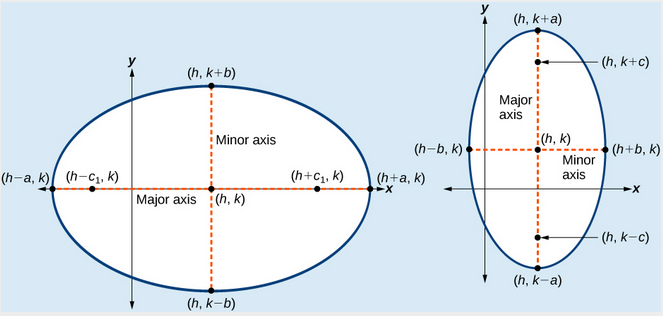(a) Horizontal ellipse with center $\left(h,k\right)$ (b) Vertical ellipse with center $\left(h,k\right)$

### How To: Given the vertices and foci of an ellipse not centered at the origin, write its equation in standard form.

1. Determine whether the major axis is parallel to the x– or y-axis.
1. If the y-coordinates of the given vertices and foci are the same, then the major axis is parallel to the x-axis. Use the standard form $\dfrac{{\left(x-h\right)}^{2}}{{a}^{2}}+\dfrac{{\left(y-k\right)}^{2}}{{b}^{2}}=1$.
2. If the x-coordinates of the given vertices and foci are the same, then the major axis is parallel to the y-axis. Use the standard form $\dfrac{{\left(x-h\right)}^{2}}{{b}^{2}}+\dfrac{{\left(y-k\right)}^{2}}{{a}^{2}}=1$.
2. Identify the center of the ellipse $\left(h,k\right)$ using the midpoint formula and the given coordinates for the vertices.
3. Find ${a}^{2}$ by solving for the length of the major axis, $2a$, which is the distance between the given vertices.
4. Find ${c}^{2}$ using $h$ and $k$, found in Step 2, along with the given coordinates for the foci.
5. Solve for ${b}^{2}$ using the equation ${c}^{2}={a}^{2}-{b}^{2}$.
6. Substitute the values for $h,k,{a}^{2}$, and ${b}^{2}$ into the standard form of the equation determined in Step 1.

### Example: Writing the Equation of an Ellipse Centered at a Point Other Than the Origin

What is the standard form equation of the ellipse that has vertices $\left(-2,-8\right)$ and $\left(-2,\text{2}\right)$ and foci $\left(-2,-7\right)$ and $\left(-2,\text{1}\right)?$

### Try It

What is the standard form equation of the ellipse that has vertices $\left(-3,3\right)$ and $\left(5,3\right)$ and foci $\left(1 - 2\sqrt{3},3\right)$ and $\left(1+2\sqrt{3},3\right)?$

## Solving Applied Problems Involving Ellipses

Many real-world situations can be represented by ellipses, including orbits of planets, satellites, moons and comets, and shapes of boat keels, rudders, and some airplane wings. A medical device called a lithotripter uses elliptical reflectors to break up kidney stones by generating sound waves. Some buildings, called whispering chambers, are designed with elliptical domes so that a person whispering at one focus can easily be heard by someone standing at the other focus. This occurs because of the acoustic properties of an ellipse. When a sound wave originates at one focus of a whispering chamber, the sound wave will be reflected off the elliptical dome and back to the other focus. In the whisper chamber at the Museum of Science and Industry in Chicago, two people standing at the foci—about 43 feet apart—can hear each other whisper.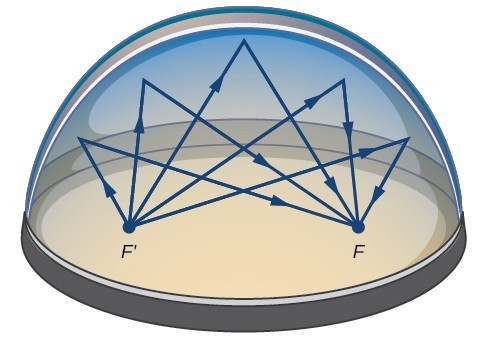### Example: Locating the Foci of a Whispering Chamber

The Statuary Hall in the Capitol Building in Washington, D.C. is a whispering chamber. Its dimensions are 46 feet wide by 96 feet long.

a. What is the standard form of the equation of the ellipse representing the outline of the room? Hint: assume a horizontal ellipse, and let the center of the room be the point $\left(0,0\right)$.

b. If two senators standing at the foci of this room can hear each other whisper, how far apart are the senators? Round to the nearest foot.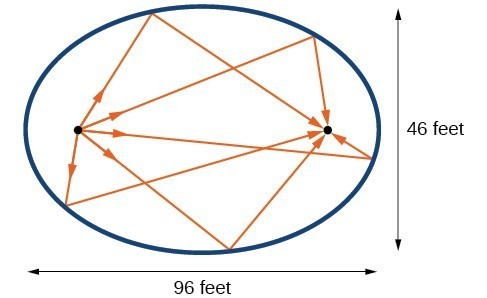### Try It

Suppose a whispering chamber is 480 feet long and 320 feet wide.

a. What is the standard form of the equation of the ellipse representing the room? Hint: assume a horizontal ellipse, and let the center of the room be the point $\left(0,0\right)$.

b. If two people are standing at the foci of this room and can hear each other whisper, how far apart are the people? Round to the nearest foot.

## Contribute!

Did you have an idea for improving this content? We’d love your input.